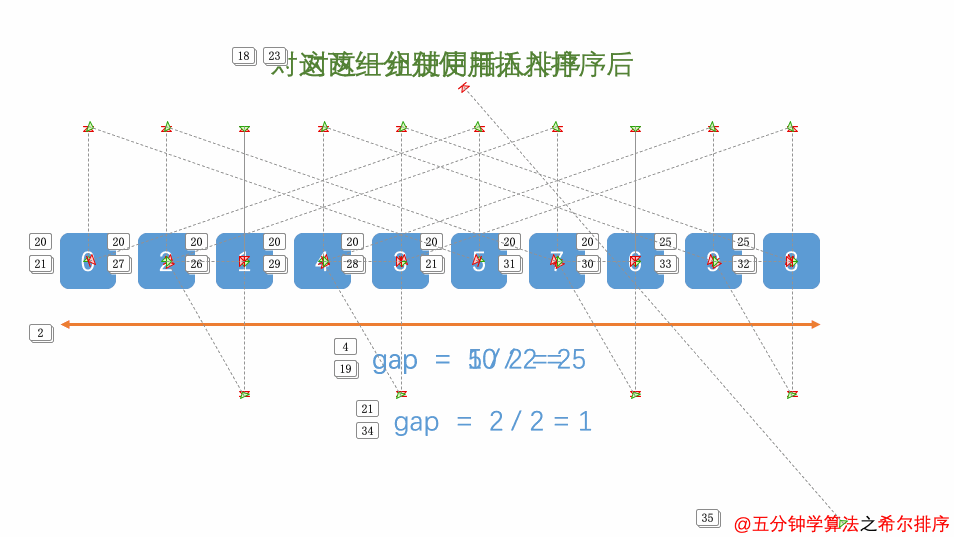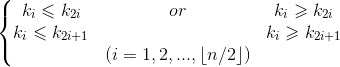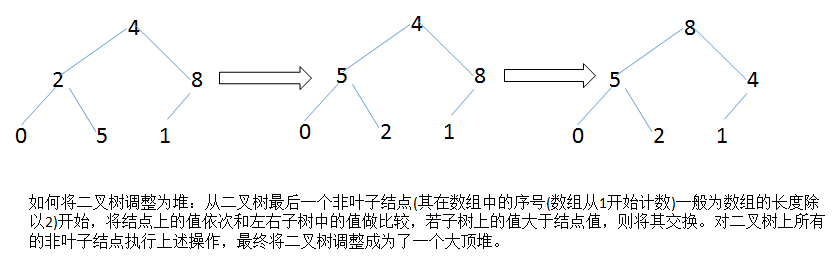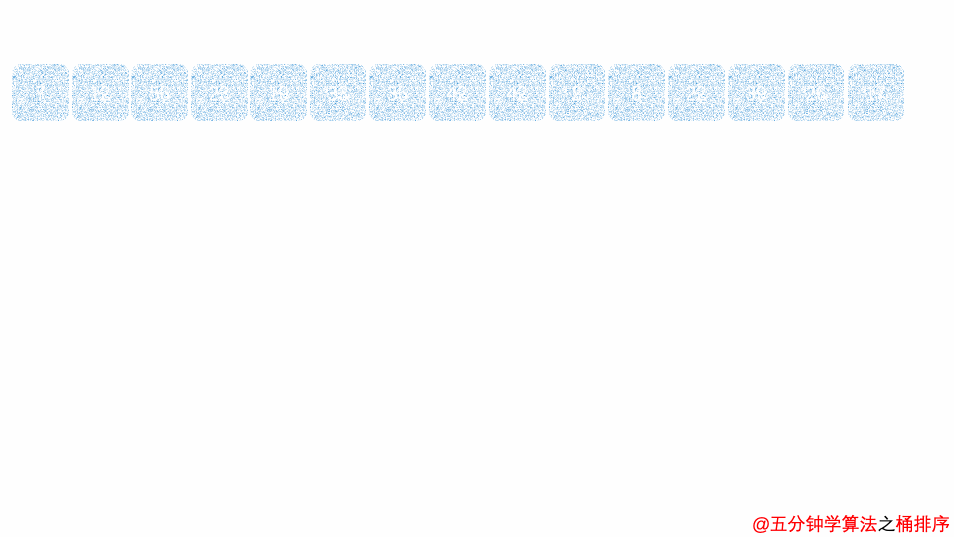# 十大经典排序算法总结（Java实现+动画）

### 0、排序算法的说明

0.1 排序的定义

0.2术语说明

• 稳定：如果a原本在b前面，而a=b,排序之后a仍然在b的前面；
• 不稳定：如果a原本在b前面，而a=b,排序之后a有可能会出现在b的后面；
• 内排序：所有排序操作都在内存中完成；
• 外排序：由于数据太大，因此把数据放在磁盘中，而排序通过磁盘和内存的数据传输才能进行；
• 时间复杂度：描述算法运行时间的函数，用大O符号表述；
• 空间复杂度：描述算法所需要的内存空间大小。

0.3算法总结n:数据规模

k:"桶"的个数

In-place:占用常数内存，不占用额外内存

Out-place:占用额外内存

0.5 算法分类0.6 比较和非比较排序的区别

1、冒泡排序

1.1 算法描述

• 比较相邻的元素。如果第一个比第二个大，就交换它们两个；
• 对每一对相邻元素作同样的工作，从开始第一对到结尾的最后一对，这样在最后的元素应该会是最大的数；
• 针对所有的元素重复以上的步骤，除了最后一个；
• 重复步骤1~3，直到排序完成。

1.2 动图演示1.3代码实现

	/**
* 冒泡排序
* @param array
* @return
*/
public static int[] bubbleSort(int[] array){
if(array.length > 0){
for(int i = 0;i<array.length;i++){
for(int j = 0;j<array.length - 1 - i;j++){
if(array[j] > array[j+1]){
int temp = array[j];
array[j] = array[j+1];
array[j+1] = temp;
}
}
}
}
return array;
}

1.4 算法分析

### 2、选择排序（Selection Sort）

2.1 算法描述

n个记录的直接选择排序可经过n-1趟直接选择排序得到有序结果。具体算法描述如下：

• 初始状态：无序区为R[1..n]，有序区为空；
• 第i趟排序(i=1,2,3…n-1)开始时，当前有序区和无序区分别为R[1..i-1]和R(i..n）。该趟排序从当前无序区中-选出关键字最小的记录 R[k]，将它与无序区的第1个记录R交换，使R[1..i]和R[i+1..n)分别变为记录个数增加1个的新有序区和记录个数减少1个的新无序区；
• n-1趟结束，数组有序化了。

2.2 动图演示2.3 代码实现

	/**
* 选择排序
* @param array
* @return
*/
public static int[] selectionSort(int[] array){
if(array.length > 0){
for(int i = 0;i<array.length;i++){
int minIndex = i;
for(int j = i;j<array.length;j++){//遍历未剩余未排序元素中继续寻找最小元素
if(array[j] < array[minIndex]){
minIndex = j;
}
}
if(minIndex != i){
int temp = array[minIndex];
array[minIndex] = array[i];
array[i] = temp;
}
}

}
return array;
}

2.4 算法分析

### 3、插入排序（Insertion Sort）

3.1 算法描述

• 从第一个元素开始，该元素可以认为已经被排序；
• 取出下一个元素，在已经排序的元素序列中从后向前扫描；
• 如果该元素（已排序）大于新元素，将该元素移到下一位置；
• 重复步骤3，直到找到已排序的元素小于或者等于新元素的位置；
• 将新元素插入到该位置后；
• 重复步骤2~5。

3.2 动图演示3.2 代码实现

	/**
* 插入排序
* @param array
* @return
*/
public static int[] insertSort(int[] array){
if(array.length > 0){
for(int i = 0 ;i<array.length - 1;i++){
int current = array[i+1];
int index = i;
while(index >= 0 && current < array[index]){
array[index + 1] = array[index];
index--;
}
array[index+1] = current;
}

}
return array;
}

3.4 算法分析

### 4、希尔排序（Shell Sort）

4.1 算法描述

• 选择一个增量序列t1，t2，…，tk，其中ti>tj，tk=1；
• 按增量序列个数k，对序列进行k 趟排序；
• 每趟排序，根据对应的增量ti，将待排序列分割成若干长度为m 的子序列，分别对各子表进行直接插入排序。仅增量因子为1 时，整个序列作为一个表来处理，表长度即为整个序列的长度。

4.2 过程演示4.3 代码实现

/**
* 希尔排序
* @param array
* @return
*/
public static int[] shellSort(int[] array){

if(array.length > 0){
int len = array.length;
int gap = len / 2;
while(gap > 0){
for(int i = gap;i < len;i++){
int temp = array[i];
int index = i - gap;
while(index >= 0 && array[index] > temp){
array[index + gap] = array[index];
index -= gap;
}
array[index + gap] = temp;
}
gap /= 2;
}

}
return array;
}

4.4 算法分析

### 5、归并排序（Merge Sort）

5.1 算法描述

• 把长度为n的输入序列分成两个长度为n/2的子序列；
• 对这两个子序列分别采用归并排序；
• 将两个排序好的子序列合并成一个最终的排序序列。

5.2 动图演示5.3 代码实现

	/**
* 2路归并算法
* @param array
* @return
*/
public static int[] MergeSort(int[] array){
if(array.length < 2){
return array;
}
int mid = array.length /2;
int[] left = Arrays.copyOfRange(array, 0, mid);
int[] right = Arrays.copyOfRange(array, mid, array.length);
return merge(MergeSort(left),MergeSort(right));
}

public static int[] merge(int[] left,int[] right){
int[] result = new int[left.length + right.length];
for(int index = 0,i = 0, j = 0;index < result.length;index++){
if(i >= left.length){
result[index] = right[j++];
}else if(j >= right.length){
result[index] = left[i++];
}else if(left[i] > right[j]){
result[index] = right[j++];
}else{
result[index] = left[i++];
}
}
return result;

}

5. 4 算法分析

### 6、快速排序（Quick Sort）

6.1 算法描述

• 从数列中挑出一个元素，称为 “基准”（pivot）；
• 重新排序数列，所有元素比基准值小的摆放在基准前面，所有元素比基准值大的摆在基准的后面（相同的数可以到任一边）。在这个分区退出之后，该基准就处于数列的中间位置。这个称为分区（partition）操作；
• 递归地（recursive）把小于基准值元素的子数列和大于基准值元素的子数列排序。

6.2 动图演示6.3 代码实现

	/**
* 快速排序算法
* @param array
* @param low
* @param hight
*/
public static void QuickSort(int[] array,int low,int hight){
//if (array.length < 1 || low < 0 || hight >= array.length || low > hight) return null;
if(low < hight){
int privotpos = partition(array,low,hight);
QuickSort(array,low,privotpos - 1);
QuickSort(array,privotpos + 1,hight);
}

}

public static int partition(int[] array,int low,int hight){
int privot = array[low];
while(low < hight){
while(low < hight && array[hight] >= privot) --hight;
array[low] = array[hight];
while(low < hight && array[low] <= privot) ++low;
array[hight] = array[low];
}
array[low] = privot;
return low;
}

6.4 算法分析

### 7、堆排序（Heap Sort）7.1 算法描述

• 将初始待排序关键字序列(R1,R2….Rn)构建成大顶堆，此堆为初始的无序区；
• 将堆顶元素R与最后一个元素R[n]交换，此时得到新的无序区(R1,R2,……Rn-1)和新的有序区(Rn),且满足R[1,2…n-1]<=R[n]；
• 由于交换后新的堆顶R可能违反堆的性质，因此需要对当前无序区(R1,R2,……Rn-1)调整为新堆，然后再次将R与无序区最后一个元素交换，得到新的无序区(R1,R2….Rn-2)和新的有序区(Rn-1,Rn)。不断重复此过程直到有序区的元素个数为n-1，则整个排序过程完成。

7.2 动图演示7.3 代码实现

	/**
* 调整堆
* @param array
* @param index
* @param length
*/
public static void heapAdjust(int[] array,int index,int length){
//保存当前结点的下标
int max = index;
//当前节点左子节点的下标
int lchild = 2*index;
//当前节点右子节点的下标
int rchild = 2*index + 1;
if(length > lchild && array[max] < array[lchild]){
max = lchild;
}
if(length > rchild && array[max] < array[rchild]){
max = rchild;
}
//若此节点比其左右孩子的值小，就将其和最大值交换，并调整堆
if(max != index){
int temp = array[index];
array[index] = array[max];
array[max] = temp;
}

}

/**
* 堆排序
* @param array
* @return
*/
public static int[] heapSort(int[] array){
int len = array.length;
//初始化堆，构造一个最大堆
for(int i = (len/2 - 1);i >= 0;i--){
}
//将堆顶的元素和最后一个元素交换，并重新调整堆
for(int i = len - 1;i > 0;i--){
int temp = array[i];
array[i] = array;
array = temp;

}
return array;
}

7.4 算法分析

### 8、计数排序（Counting Sort）

8.1 算法描述

• 找出待排序的数组中最大和最小的元素；
• 统计数组中每个值为i的元素出现的次数，存入数组C的第i项；
• 对所有的计数累加（从C中的第一个元素开始，每一项和前一项相加）；
• 反向填充目标数组：将每个元素i放在新数组的第C(i)项，每放一个元素就将C(i)减去1。

8.2 动图演示8.3 代码实现

	/**
* 计数排序
* @param array
* @return
*/
public static int[] countingSort(int[] array){
if(array.length == 0){
return array;
}
int bias ,min = array,max = array;
//找出最小值和最大值
for(int i = 0;i < array.length;i++){
if(array[i] < min){
min = array[i];
}
if(array[i] > max){
max = array[i];
}
}
//偏差
bias = 0 - min;
//新开辟一个数组
int[] bucket = new int[max - min +1];
//数据初始化为0
Arrays.fill(bucket, 0);
for(int i = 0;i < array.length;i++){
bucket[array[i] + bias] += 1;
}

int index = 0;
for(int i = 0;i < bucket.length;i++){
int len = bucket[i];
while(len > 0){
array[index++] = i - bias;
len --;
}
}

return array;

}

8.4 算法分析

### 9、桶排序（Bucket Sort）

9.1 算法描述

• 人为设置一个BucketSize，作为每个桶所能放置多少个不同数值（例如当BucketSize==5时，该桶可以存放｛1,2,3,4,5｝这几种数字，但是容量不限，即可以存放100个3）；
• 遍历输入数据，并且把数据一个一个放到对应的桶里去；
• 对每个不是空的桶进行排序，可以使用其它排序方法，也可以递归使用桶排序；
• 从不是空的桶里把排好序的数据拼接起来。

9.2 图片演示9.3 代码实现

	/**
* 桶排序
*
* @param array
* @param bucketSize 桶中可以放多少种元素
* @return
*/
public static ArrayList<Integer> BucketSort(ArrayList<Integer> array, int bucketSize) {
if (array == null || array.size() < 2)
return array;
int max = array.get(0), min = array.get(0);
// 找到最大值最小值
for (int i = 0; i < array.size(); i++) {
if (array.get(i) > max)
max = array.get(i);
if (array.get(i) < min)
min = array.get(i);
}
int bucketCount = (max - min) / bucketSize + 1;
ArrayList<ArrayList<Integer>> bucketArr = new ArrayList<>(bucketCount);
ArrayList<Integer> resultArr = new ArrayList<>();
//构造桶
for (int i = 0; i < bucketCount; i++) {
}
//往桶里塞元素
for (int i = 0; i < array.size(); i++) {
}
for (int i = 0; i < bucketCount; i++) {
if (bucketSize == 1) {
for (int j = 0; j < bucketArr.get(i).size(); j++)
} else {
if (bucketCount == 1)
bucketSize--;
ArrayList<Integer> temp = BucketSort(bucketArr.get(i), bucketSize);
for (int j = 0; j < temp.size(); j++)
}
}
return resultArr;
}

9.4 算法分析

10.1 算法描述

• 取得数组中的最大数，并取得位数；

10.2 动图演示10.3 代码实现

	/**
* 基数排序
* @param array
* @return
*/
public static int[] RadixSort(int[] array) {
if (array == null || array.length < 2)
return array;
// 1.先算出最大数的位数；
int max = array;
for (int i = 1; i < array.length; i++) {
max = Math.max(max, array[i]);
}
int maxDigit = 0;
while (max != 0) {
max /= 10;
maxDigit++;
}
int mod = 10, div = 1;
ArrayList<ArrayList<Integer>> bucketList = new ArrayList<ArrayList<Integer>>();
for(int i = 0; i < 10;i++){
}
for(int i = 0;i < maxDigit;i++,mod *= 10 ,div *= 10){
for(int j = 0;j < array.length;j++){
int num = (array[j] % mod) / div;
}
int index = 0;
for(int j = 0;j < bucketList.size();j++){
for(int k = 0;k < bucketList.get(j).size();k++){
array[index++] = bucketList.get(j).get(k);
}
bucketList.get(j).clear();
}
}

return array;
}

10.4 算法分析

MSD 从高位开始进行排序 LSD 从低位开始进行排序

• 基数排序：根据键值的每位数字来分配桶
• 计数排序：每个桶只存储单一键值
• 桶排序：每个桶存储一定范围的数值

09-25339
03-223374
08-293307
12-2761
08-222万+
07-23103万+
04-167716
04-171万+
11-052万+
03-067251
01-221010
02-13398
09-21
09-24
03-06
10-231万+
10-29311
05-185513
10-137307
03-07

### “相关推荐”对你有帮助么？

•非常没帮助
•没帮助
•一般
•有帮助
•非常有帮助被折叠的  条评论 为什么被折叠?到【灌水乐园】发言meibenxiang

¥2 ¥4 ¥6 ¥10 ¥20余额支付 (余额：-- )扫码支付获取中扫码支付点击重新获取扫码支付1.余额是钱包充值的虚拟货币，按照1:1的比例进行支付金额的抵扣。
2.余额无法直接购买下载，可以购买VIP、C币套餐、付费专栏及课程。余额充值